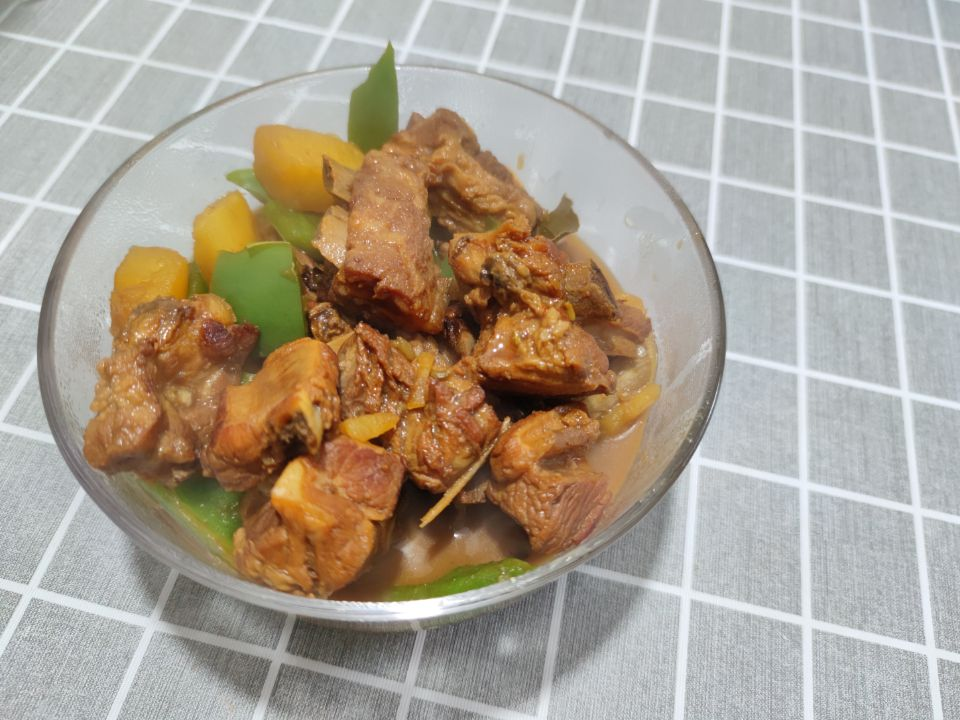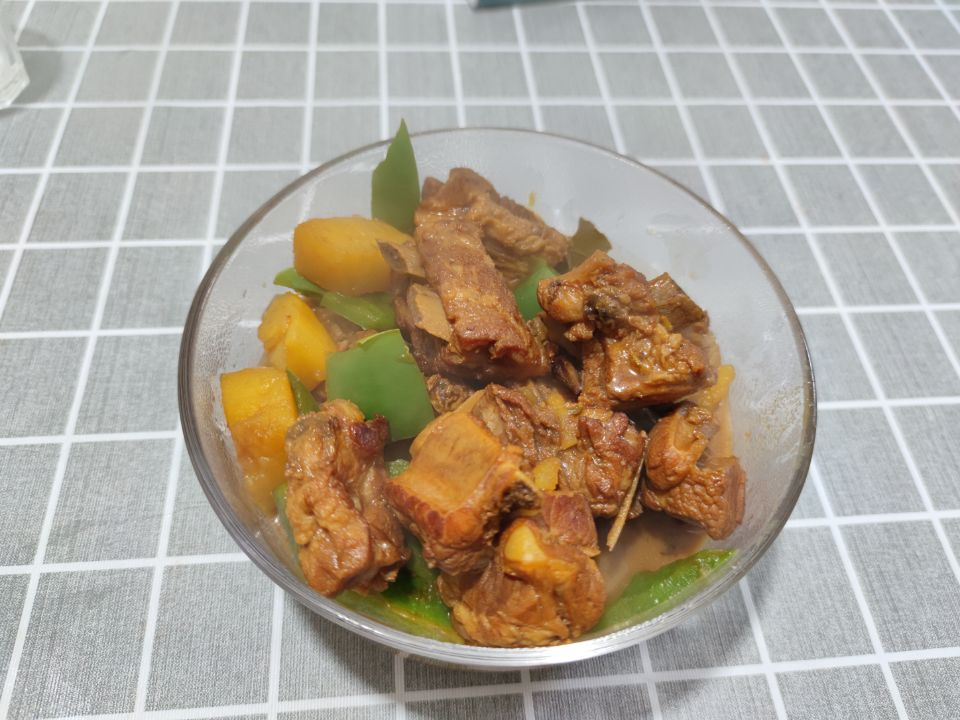# 土豆炖排骨的做法

• 肋排
• 土豆
• 小葱
• 料酒
• 白糖
• 干辣椒
• 八角
• 花椒
• 桂皮
• 生抽
• 老抽
• 蚝油
• 黄豆酱

• 肋排 = 750g
• 土豆 = 300g
• 姜 = 30g
• 小葱 = 25g
• 料酒 = 25g
• 白糖 = 10g
• 干辣椒 = 5g
• 八角 = 5g
• 花椒 = 5g
• 桂皮 = 5g
• 生抽 = 10g
• 老抽 = 5g
• 蚝油 = 5g
• 黄豆酱 = 5g

## 操作

• 土豆两个滚刀切片，姜片切片
• 排骨 750g 冷水下锅，加入姜片、葱段、料酒焯水 2 分钟，焯干水后捞出清洗干净（一定要用热水，不能用冷水）
• 热锅凉油，将白糖倒入锅中，翻炒至融化为焦糖色
• 加入排骨煎至两面金黄，让排骨裹满焦糖
• 加入干辣椒、八角、花椒、桂皮、姜片（建议买超市的香料包）、10ml 生抽、5ml 老抽、5ml 料酒、5ml 蚝油、5ml 黄豆酱
• 大火翻炒均匀后加入 700ml 开水，大火烧开后转小火焖煮 1 小时
• 最后加入土豆煮 10 分钟就可以出锅啦（喜欢吃青红椒的也可以按自己喜好加入）
••